Homework Help Question & Answers

# This exercise examines the accuracy of various branch predictors for the following repeating pattern (e.g. in...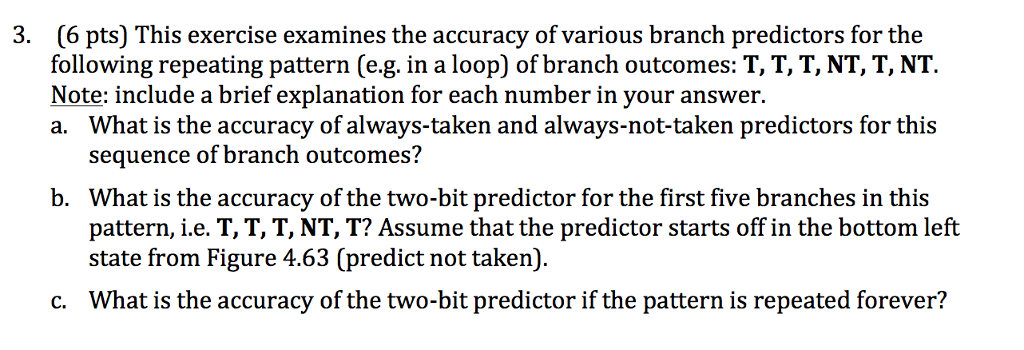This exercise examines the accuracy of various branch predictors for the following repeating pattern (e.g. in a loop) of branch outcomes: T, T, T, NT, T, NT. a. What is the accuracy of always-taken and always-not-taken predictors for this sequence of branch outcomes? b. What is the accuracy of the two-bit predictor for the first five branches in this pattern, i.e. T, T, T, NT, T? Assume that the predictor starts off in the bottom left state from Figure 4.63 (predict not taken). c. What is the accuracy of the two-bit predictor if the pattern is repeated forever?

#### Homework Answers

Answer #1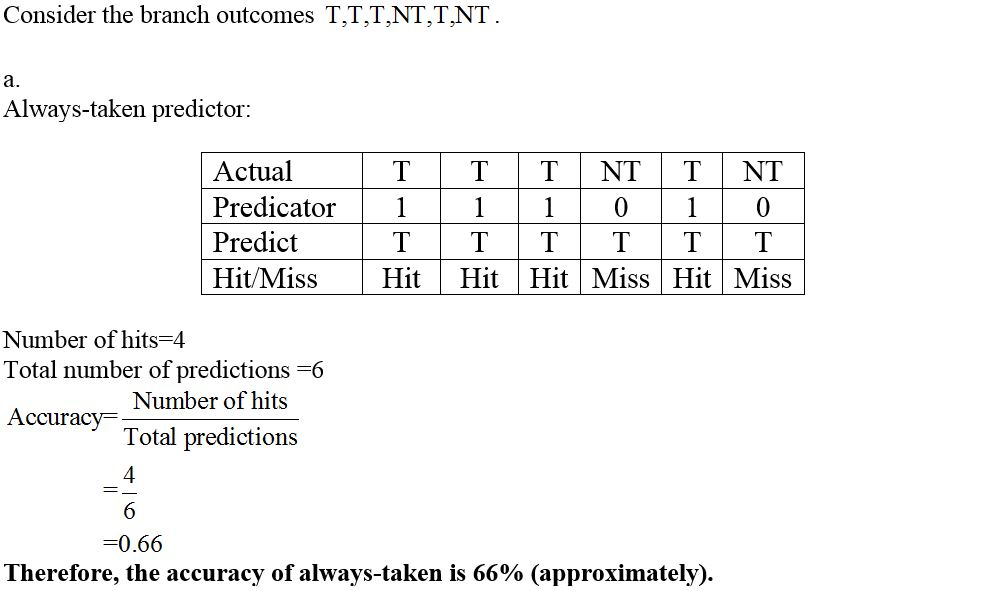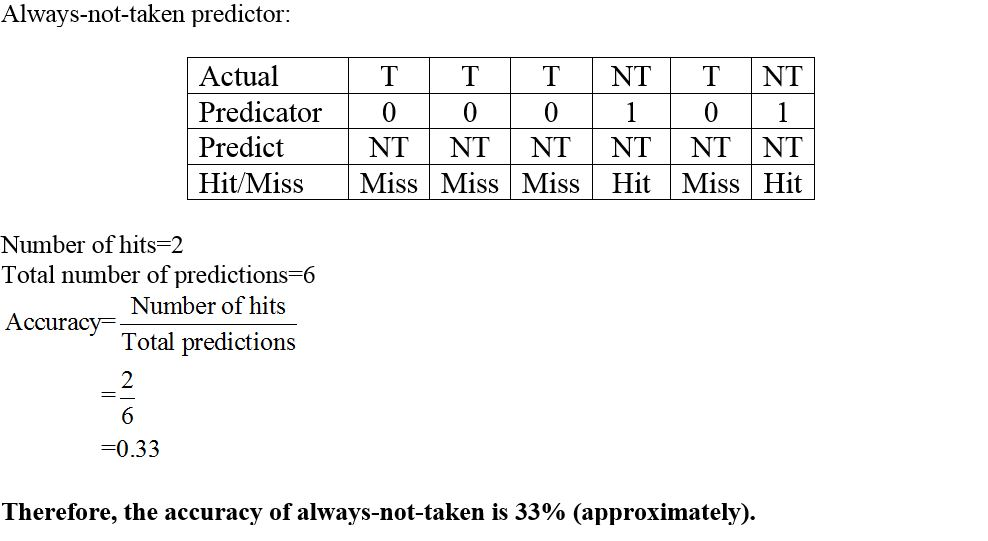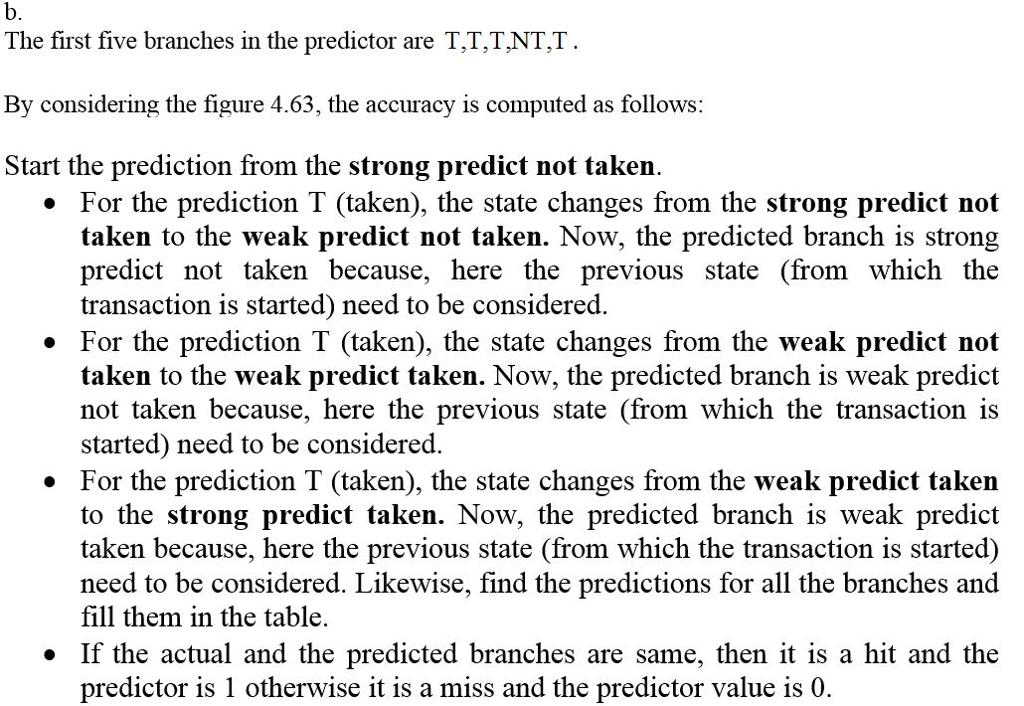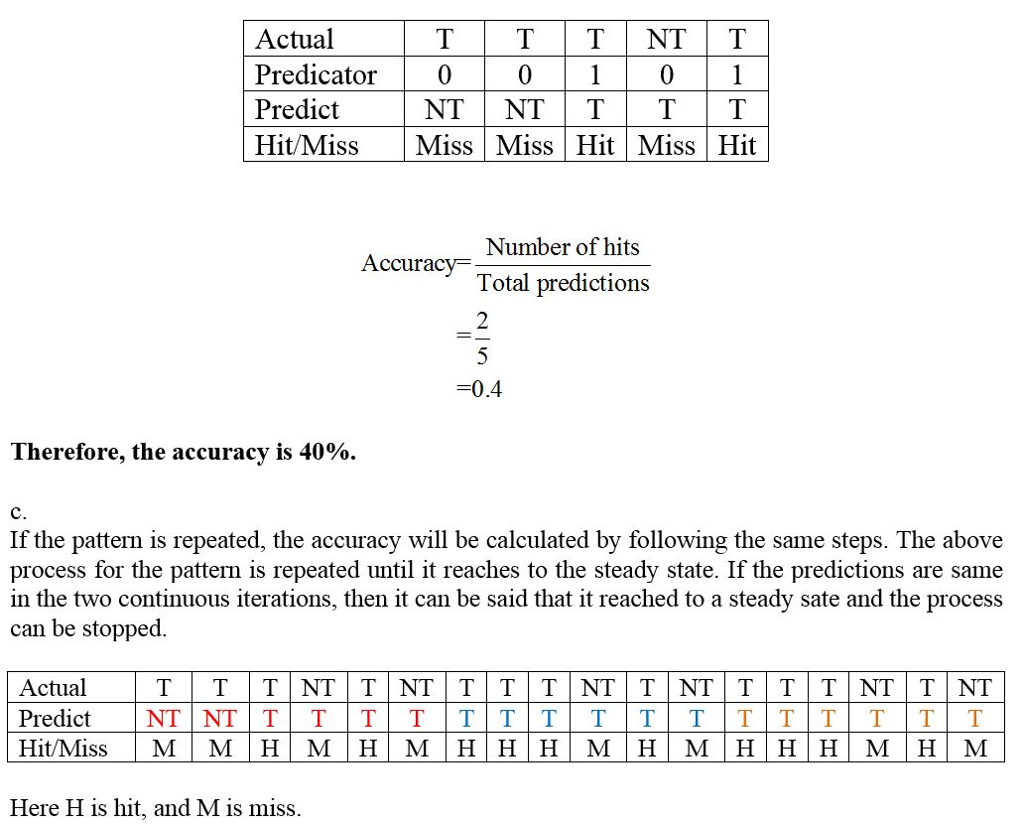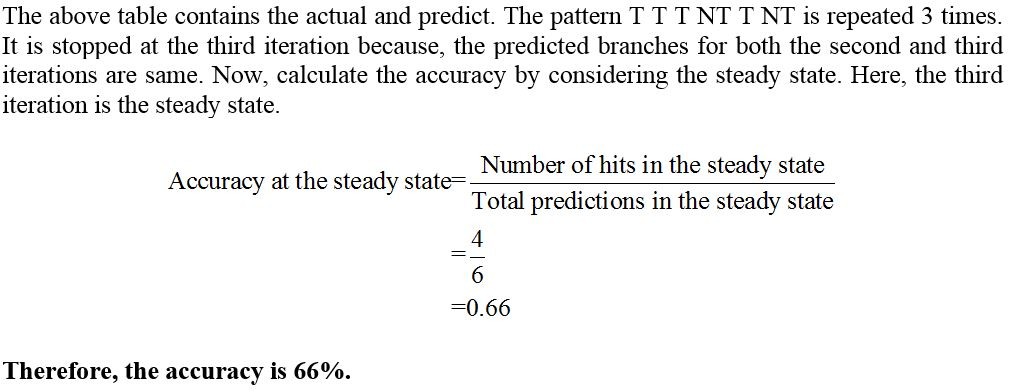Know the answer?
Your Answer:

#### Post as a guest

Your Name:

What's your source?

#### Earn Coin

Coins can be redeemed for fabulous gifts.

Not the answer you're looking for? Ask your own homework help question. Our experts will answer your question WITHIN MINUTES for Free.
Similar Homework Help Questions
• ### 14(10 points) In this problem we want to examine the accuracy of branch predictors for the...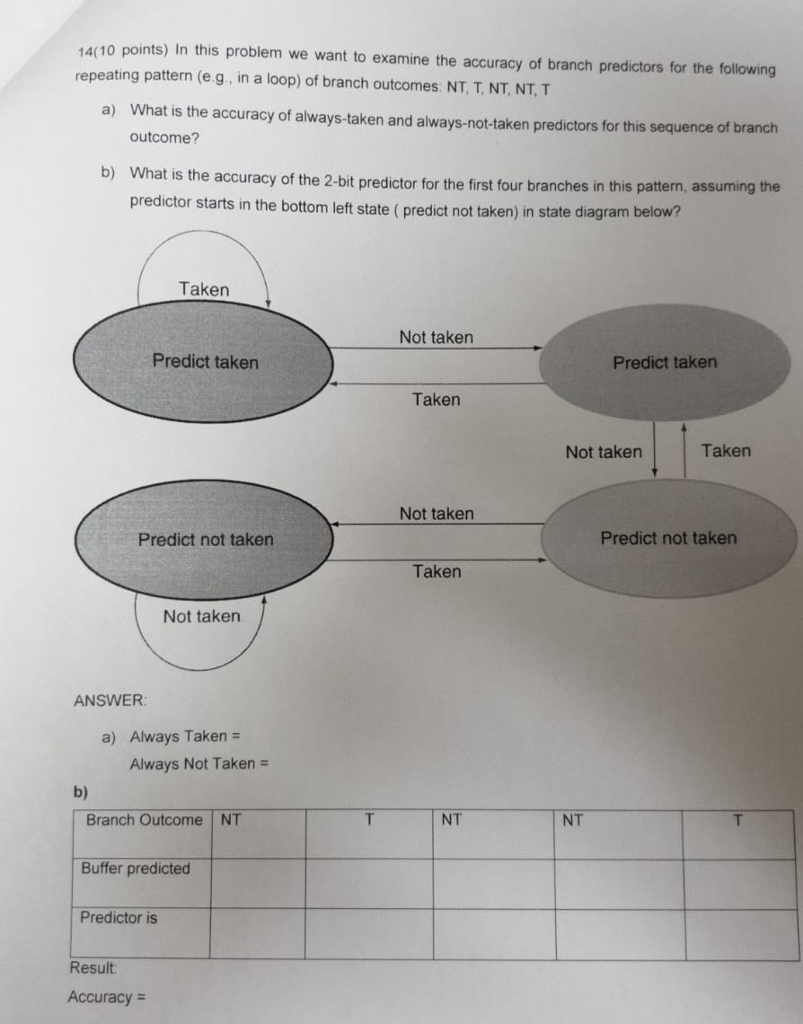14(10 points) In this problem we want to examine the accuracy of branch predictors for the following repeating pattern (e.g, in a loop) of branch outcomes: NT, T, NT, NT, T What is the accuracy of always-taken and always-not-taken predictors for this sequence of branch outcome? a) b) Wh at is the accuracy of the 2-bit predictor for the first four branches in this pattern, assuming the predictor starts in the bottom left state ( predict not taken) in state...

• ### 1. Suppose we have a 5-stage pipeline CPU and run the following instructions: or \$tl, \$t2, \$t3 or...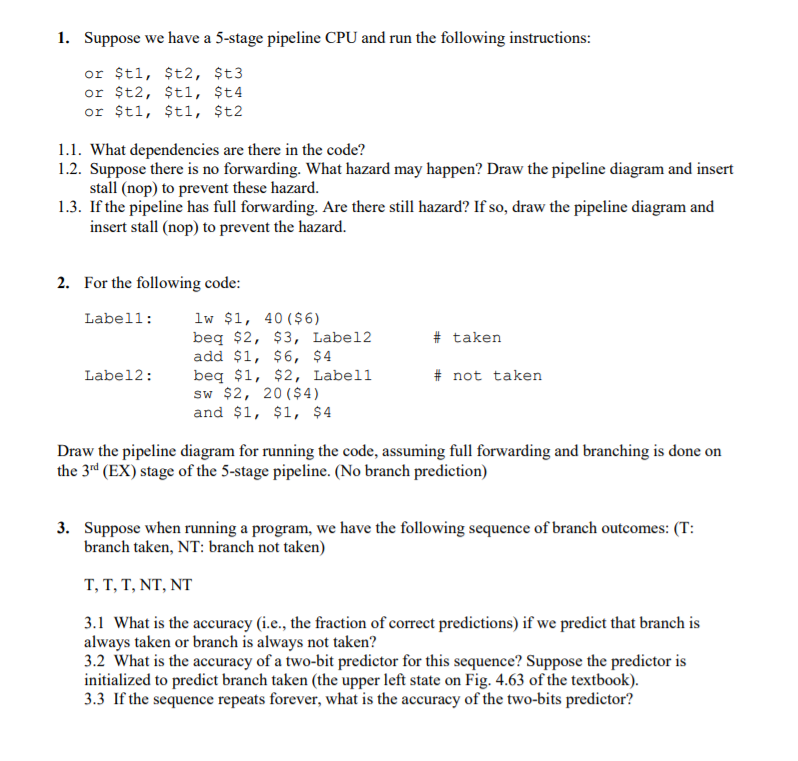1. Suppose we have a 5-stage pipeline CPU and run the following instructions: or \$tl, \$t2, \$t3 or \$t2, \$tl, \$t4 or \$tl, \$tl, \$t2 1.1. What dependencies are there in the code? 1.2. Suppose there is no forwarding. What hazard may happen? Draw the pipeline diagram and insert stall (nop) to prevent these hazard. 1.3. If the pipeline has full forwarding. Are there still hazard? If so, draw the pipeline diagram and insert stall (nop) to prevent the hazard....

• ### Ch04.4. [6 points] Branch Prediction. Consider the following sequence of actual outcomes for a si...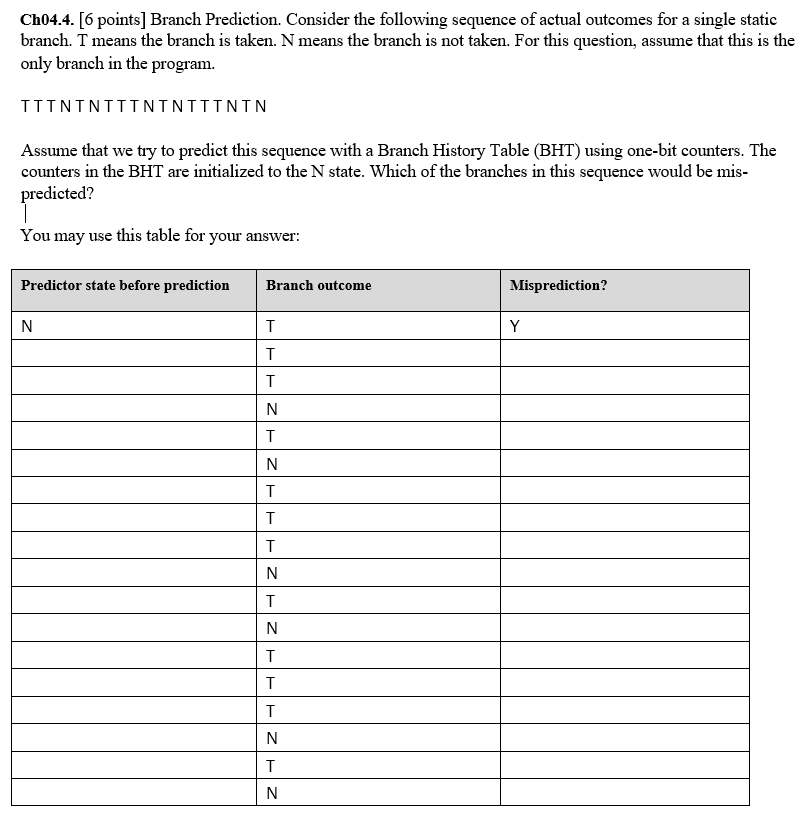Ch04.4. [6 points] Branch Prediction. Consider the following sequence of actual outcomes for a single static branch. T means the branch is taken. N means the branch is not taken. For this question, assume that this is the only branch in the program. TTTNTNTTTNTNTTTNTN Assume that we try to predict this sequence with a Branch History Table (BHT) using one-bit counters. The counters in the BHT are initialized to the N state. Which of the branches in this sequence would...

• ### The following sequence of branch outcomes is applied to a saturating counter branch predictor T T...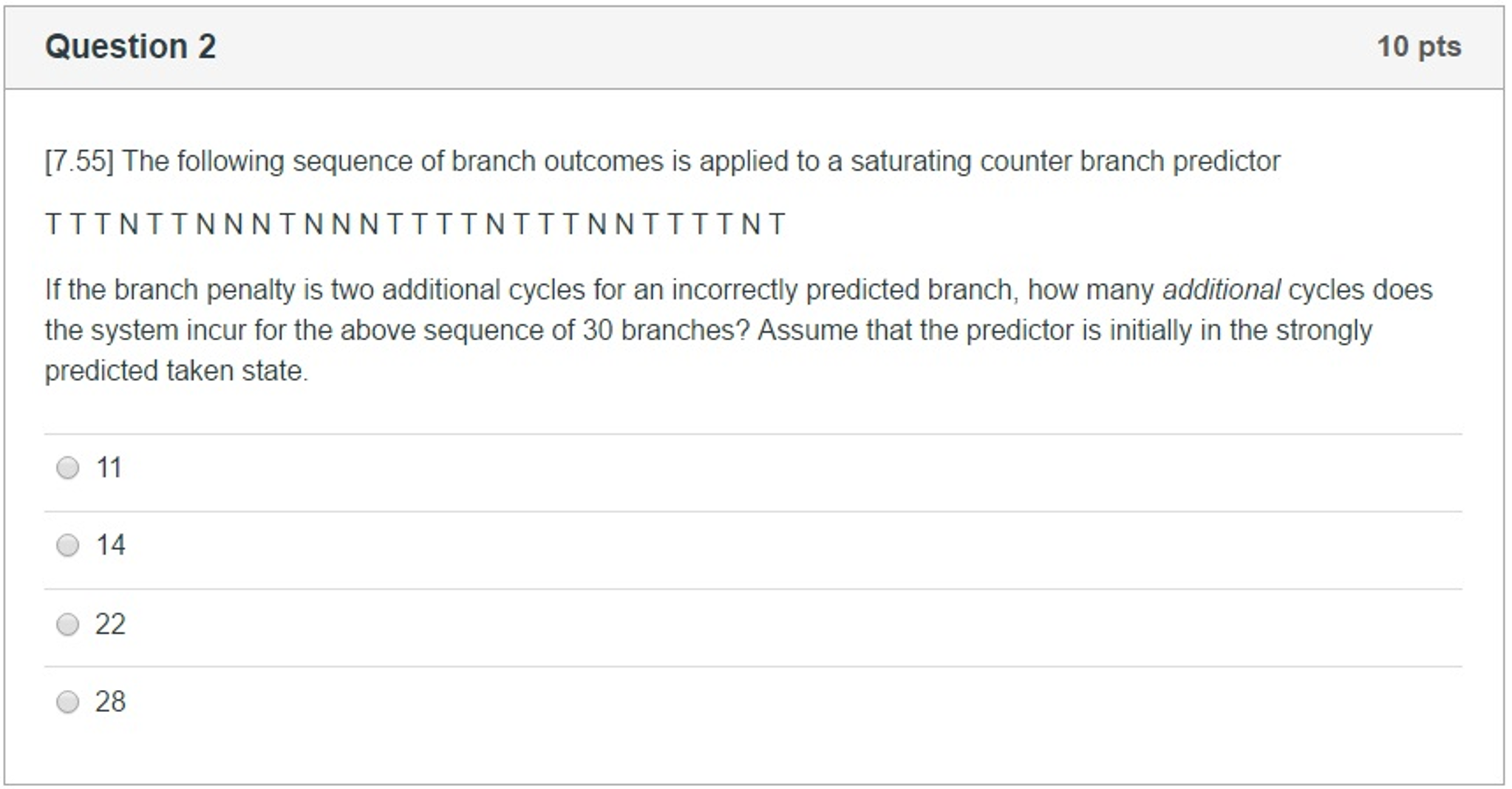The following sequence of branch outcomes is applied to a saturating counter branch predictor T T T N T T N N N T N N N T T T T N T T T N N T T T T N T If the branch penalty is two additional cycles for an incorrectly predicted branch, how many additional cycles does the system incur for the above sequence of 30 branches? Assume that the predictor is initially in the strongly...

Free Homework App

Scan Your Homework
to Get Instant Free Answers
Need Online Homework Help?

Get Answers For Free
Most questions answered within 3 hours.